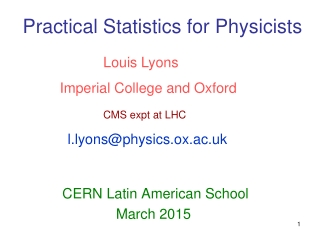DownloadDownload PresentationPractical Statistics for Physicists

# Practical Statistics for Physicists

Télécharger la présentation## Practical Statistics for Physicists

- - - - - - - - - - - - - - - - - - - - - - - - - - - E N D - - - - - - - - - - - - - - - - - - - - - - - - - - -
##### Presentation Transcript

1. Louis Lyons Imperial College and Oxford CMS expt at LHC l.lyons@physics.ox.ac.uk Practical Statistics for Physicists CERN Latin American School March 2015

2. Extra Lecture:Learning to love the Error Matrix • Introduction via 2-D Gaussian • Understanding covariance • Using the error matrix Combining correlated measurements • Estimating the error matrix

3. y = 1 exp{-(x-µ)2/(22)} 2  Reminder of 1-D Gaussian or Normal

4. Correlations Basic issue: For 1 parameter, quote value and error For 2 (or more) parameters, (e.g. gradient and intercept of straight line fit) quote values + errors + correlations Just as the concept of variance for single variable is more general than Gaussian distribution, so correlation in more variables does not require multi-dim Gaussian But more simple to introduce concept this way

5. Element Eij - <(xi – xi) (xj – xj)> Diagonal Eij = variances Off-diagonal Eij = covariances

6. Towards the Error Matrix

7. Example from Particle Physics

8. Examples of correlated variables

9. Small error Example: Chi-sq Lecture xbest outside x1 x2 ybest outside y1  y2

10. b y a x

11. Conclusion Error matrix formalism makes life easy when correlations are relevant